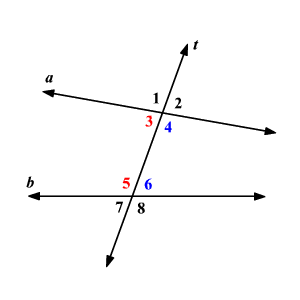# Consecutive Interior Angles Theorem

### Consecutive Interior Angles

When two lines are cut by a transversal, the pair of angles on one side of the transversal and inside the two lines are called the consecutive interior angles.In the figure, the angles $3$ and $5$ are consecutive interior angles.

Also the angles $4$ and $6$ are consecutive interior angles.

### Consecutive Interior Angles Theorem

If two parallel lines are cut by a transversal, then the pairs of consecutive interior angles formed are supplementary.

Proof:

Given: $k\parallel l$ , $t$ is a transversal

Prove: $\angle 3$ and $\angle 5$ are supplementary and $\angle 4$ and $\angle 6$ are supplementary.

 Statement Reason 1 $k\parallel l$ , $t$ is a traversal. Given 2 $\angle 1$ and $\angle 3$ form a linear pair and $\angle 2$ and $\angle 4$ form a linear pair. Definition of linear pair 3 $\angle 1$ and $\angle 3$ are supplementary $m\angle 1+m\angle 3=180°$ $\angle 2$ and $\angle 4$ are supplementary $m\angle 2+m\angle 4=180°$ 4 $\angle 1\cong \angle 5$ and $\angle 2\cong \angle 6$ Corresponding Angles Theorem 5 $\angle 3$ and $\angle 5$ are supplementary $\angle 4$ and $\angle 6$ are supplementary. Substitution Property